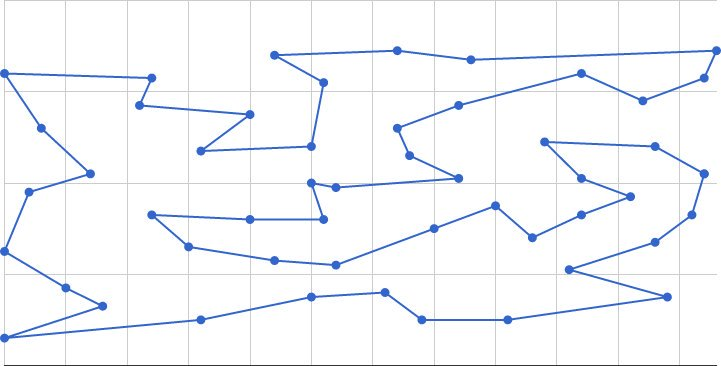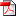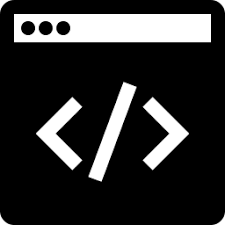MAOS-TSP  is a multiagent optimization system (MAOS) for solving the Traveling Salesman Problem (TSP). The source code can be downloaded here.

Related Information: Please find other related code and software in our Source Code Library.

Basic Description What’s New Directories & Files Command Line & Parameters Output Information References ContactLicense Information: You can redistribute it and/or modify it under the terms of the Creative Commons Non-Commercial License 3.0.

System Requirements: MAOS-TSP is a platform-independent software developed by JAVA version 1.5 or above.

What’s New

[Jul 27, 2015]: Add a new parameter “opt=[value]” to provide the length of the shortest tour as another stopping criterion. (Based on the request by Jakob Bossek)

– Solver “STD_PRB_3Opt_2L_REAX+SEAX_1J”: 1) Two-level representation for 3-opt: reduce the time complexity of Tour Segment Inversion from O(n) to O(sqrt(n)); 2) Locking common edges during the sub-cycles bridging process in the generalized EAX; 3) Sorting in k Nearest Neighbors: reduce the time complexity from O(nlog(n)) to O(n+klog(k)).

– Solver “STD_PRB_3Opt_REAX+SEAX”: It implements the solver for original MAOS-TSP algorithm . This solver has shown to be competitive in solving middle-scale TSP instances (1000 – 6000 nodes) as comparing to some state-of-the-art algorithms, without using explicit local search (e.g. LK variants) during the runtime cycles.

For example, it found the best-so-far solution (located in myprojects/tasks/TSP/solution) for the open instance fdp3256 in the VLSI Data Sets.

– Setting parameters: TSP:Problem, N, T, Tcon, Solver.

Directories & Files
```binary	                           // the binary code of MAOS-TSP
source                             // the source code of MAOS-TSP
myprojects                         // user directory
|-----> examples.bat               // commandline examples
|-----> results                    // the directory for storing runtime results
|-----> setting                    // the setting directory
|-----> kernel             // the setting directory for MAOS Kernel
|-----> TSP                // the setting directory for TSP solvers
|-----> solver     // the directory containing solver script files (the name of each file is Solver_Name)
// Examples: "STD_PRB_3Opt_REAX+SEAX" and "STD_PRB_3Opt_2L_REAX+SEAX_1J"
|-----> TSP
|-----> instance   // GCP instances of the TSPLIB format (Problem_Name with the default suffix .tsp)
// Example: rl1323.tsp (Problem_Name is rl1323)
|-----> solution   // the directory for storing solutions of TSP instances
// For a normal solution, the file name is Problem_Name.(objective value).tour
// If objective value is removed from the file name, the solution is considered as optimal.
// Example: fdp3256.tour

// Typical benchmark instances can be found in the TSPLIB and VLSI data sets.
```

Command Line & Parameters

MAOS-TSP is executed on the command line (Enter the directory “myprojects”, and execute maosKernel.MAOSExecuter). Here is a typical example (See the file “myprojects/Examples.bat” for more examples), in which a user should specify JAVA options, general parameters, problem instance, and solver instance:

```\$ cd myprojects
\$ java -server -Xmx512M -cp ../binary/MAOS_SEQ.jar maosKernel.MAOSExecuter TSP:Problem=rl1323 N=300 T=500 Tcon=100 DUP_TIMES=5 solver=STD_PRB_3Opt_2L_REAX+SEAX_1J

//java: the JAVA executor, JAVA Runtime Environment Version 1.5 or above is preferred
//maosKernel.MAOSExecuter: the main execution entrance of the MAOS program
```

```There are two recommended optional parameters for java:
-server: select the "server" VM, which is normally faster than the "client" VM
-Xmx: set maximum Java heap size. Using a large enough size may prevent from the "out of memory" error

Moreover, the "-cp" option is used for loading the binary file "../binary/MAOS_SEQ.jar".
```

General Parameters:

```NAME         VALUE_type   Range      Default_Value   Description

TSP:Problem  String      *	     <Problem_Name>  The problem instance to be solved
--------------------------------------------------------------------------------------------------
N            integer     >1          300 	     The number of agents
T            integer     >0          500	     Terminate condition: The maximum learning cycles
Tcon         integer     >0          100             Terminate condition: The number of cycles as the best state is unvaried

DUP_TIMES    integer     >0          10		     Number of trials
--------------------------------------------------------------------------------------------------
Solver       String      *	     <Solver_Name>   The name of the script of the actual solver
--------------------------------------------------------------------------------------------------
opt          integer    >0           None            The lower bound tour length for termination
```
`//The explanation of Problem_Name and Solver_Name can be found in Directories & Files.`

Output Information

For the output, we provide screen output, output a file for the running result, and stored the best solution ever found.

Screen Output:

```[Initialization information]: provide the parsing information during the initialization.
[Runtime information]: The program outputs runtime information, i.e., the current best evaluation values, execution time, at every "Tout" cycles.
[Summary information]: At the end, it outputs the input variables, response values, and evaluation values <Vcon, Vopt> of the best solution.
```

Result File:

```The result file will be stored at the directory "myprojects/results".
```

Solution File:

```The best solution (better than any previous solutions) will be stored at the directory "myprojects/tasks/TSP/solution" (of the TSPLIB format).
```
References
 “Multiagent optimization system for solving the traveling salesman problem (TSP),” IEEE Transactions on Systems, Man, and Cybernetics, Part B: Cybernetics, vol. 39, iss. 2, 2009.``````@Article{xie2009multiagent,
Title = {Multiagent optimization system for solving the traveling salesman problem ({TSP})},
Author = {Xiao-Feng Xie and Jiming Liu},
Journal = {IEEE Transactions on Systems, Man, and Cybernetics, Part B: Cybernetics},
Year = {2009},
Number = {2},
Pages = {489--502},
PDF={http://www.wiomax.com/team/xie/paper/TSMCB09.pdf},
DOI={10.1109/TSMCB.2008.2006910},
Code={http://www.wiomax.com/maos-tsp},
Volume = {39}
}``````